# Area of a trapezoid Online Quiz

Following quiz provides Multiple Choice Questions (MCQs) related to Area of a trapezoid. You will have to read all the given answers and click over the correct answer. If you are not sure about the answer then you can check the answer using Show Answer button. You can use Next Quiz button to check new set of questions in the quiz.Q 1 - Find the area of the following trapezoid.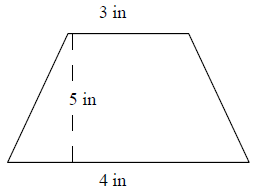### Explanation

Step 1:

Area of Trapezoid = $\frac{1}{2}$ × h × (b1 + b2); b1, b2 = bases = 3, 4; h = height = 5.

Step 2:

Area of trapezoid = $\frac{1}{2}$ × 5 × (3 + 4) = 17.5 square in

Q 2 - Find the area of the following trapezoid.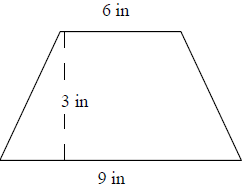### Explanation

Step 1:

Area of Trapezoid = $\frac{1}{2}$ × h × (b1 + b2); b1, b2 = bases = 6, 9; h = height = 3.

Step 2:

Area of trapezoid = $\frac{1}{2}$ × 3 × (6 + 9) = 22.5 square in

Q 3 - Find the area of the following trapezoid.### Explanation

Step 1:

Area of Trapezoid = $\frac{1}{2}$ × h × (b1 + b2); b1, b2 = bases = 3, 4.5; h = height = 5.

Step 2:

Area of trapezoid = $\frac{1}{2}$ × 5 × (3 + 4.5) = 18.75 square in

Q 4 - Find the area of the following trapezoid.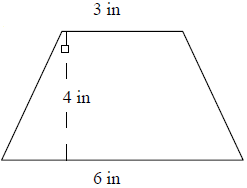### Explanation

Step 1:

Area of Trapezoid = $\frac{1}{2}$ × h × (b1 + b2); b1, b2 = bases = 3, 6; h = height = 4.

Step 2:

Area of trapezoid = $\frac{1}{2}$ × 4 × (3 + 6) = 18 square in

Q 5 - Find the area of the following trapezoid.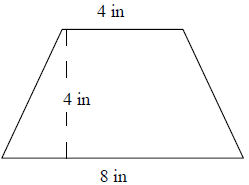### Explanation

Step 1:

Area of Trapezoid = $\frac{1}{2}$ × h × (b1 + b2); b1, b2 = bases = 4, 8; h = height = 4.

Step 2:

Area of trapezoid = $\frac{1}{2}$ × 4 × (8 + 4) = 24 square in

Q 6 - Find the area of the following trapezoid.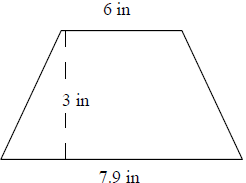### Explanation

Step 1:

Area of Trapezoid = $\frac{1}{2}$ × h × (b1 + b2); b1, b2 = bases = 6, 7.9; h = height = 3.

Step 2:

Area of trapezoid = $\frac{1}{2}$ × 3 × (6 + 7.9) = 20.85 square in

Q 7 - Find the area of the following trapezoid.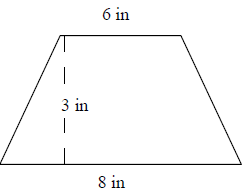### Explanation

Step 1:

Area of Trapezoid = $\frac{1}{2}$ × h × (b1 + b2); b1, b2 = bases = 6, 8; h = height = 3.

Step 2:

Area of trapezoid = $\frac{1}{2}$ × 3 × (6 + 8) = 21 square in

Q 8 - Find the area of the following trapezoid.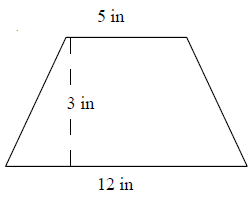### Explanation

Step 1:

Area of Trapezoid = $\frac{1}{2}$ × h × (b1 + b2); b1, b2 = bases = 5, 12; h = height = 3.

Step 2:

Area of trapezoid = $\frac{1}{2}$ × 3 × (5 + 12) = 25.5 square in

Q 9 - Find the area of the following trapezoid.### Explanation

Step 1:

Area of Trapezoid = $\frac{1}{2}$ × h × (b1 + b2); b1, b2 = bases = 5, 8; h = height = 4.4.

Step 2:

Area of trapezoid = $\frac{1}{2}$ × 4.4 × (5 + 8) = 28.6 square in

Q 10 - Find the area of the following trapezoid.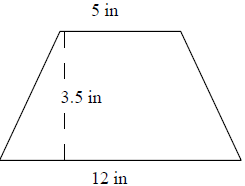### Explanation

Step 1:

Area of Trapezoid = $\frac{1}{2}$ × h × (b1 + b2); b1, b2 = bases = 5, 12; h = height = 3.5.

Step 2:

Area of trapezoid = $\frac{1}{2}$ × 3.5 × (5 + 12) = 29.75 square in

area_of_trapezoid.htm